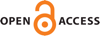Download this articleFor screen For printingRecent IssuesThe Journal About the Journal Editorial Board Editorial Interests Subscriptions Submission Guidelines Submission Page Policies for Authors Ethics Statement ISSN (electronic): 1472-2739 ISSN (print): 1472-2747 Author Index To Appear Other MSP JournalsThe upsilon invariant at $1$ of $3$–braid knots

### Paula Truöl

Algebraic & Geometric Topology 23 (2023) 3763–3804##### Abstract

We provide explicit formulas for the integer-valued smooth concordance invariant $\upsilon \left(K\right)={\mathrm{Υ}}_{K}\left(1\right)$ for every $3$–braid knot $K$. We determine this invariant, which was defined by Ozsváth, Stipsicz and Szabó (2017), by constructing cobordisms between $3$–braid knots and (connected sums of) torus knots. As an application, we show that for positive $3$–braid knots $K$ several alternating distances all equal the sum $g\left(K\right)+\upsilon \left(K\right)$, where $g\left(K\right)$ denotes the $3$–genus of $K$. In particular, we compute the alternation number, the dealternating number and the Turaev genus for all positive $3$–braid knots. We also provide upper and lower bounds on the alternation number and dealternating number of every $3$–braid knot which differ by $1$.

##### Keywords
knots, concordance, $3$–braids, upsilon invariant, alternation number, fractional Dehn twist coefficient
##### Mathematical Subject Classification
Primary: 57K10
Secondary: 20F36, 57K18
##### Publication